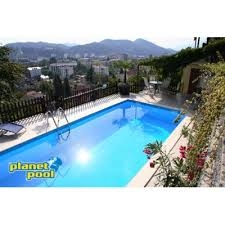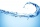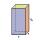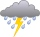# Three-quarters of its volume

The pool has a block shape with a length of 8m, a width of 5.3m and a depth of 1.5m. How many hl of water is in it if it is filled to three-quarters of its volume?

Correct result:

V =  477 hl

#### Solution:We would be pleased if you find an error in the word problem, spelling mistakes, or inaccuracies and send it to us. Thank you!Tips to related online calculators
Do you know the volume and unit volume, and want to convert volume units?

#### You need to know the following knowledge to solve this word math problem:

We encourage you to watch this tutorial video on this math problem:

## Next similar math problems:

• The shopThe shop has 3 hectoliters of water. How many liter bottles is it?
• Cargo spaceThe cargo space of the truck has the dimensions a = 4.2 m, b = 1.9 m, c = 8.1 dm. Calculate its volume.
• A swimingA swiming pool holds 30000lt of water. How many gallons does it hold? 1 gallon= 4.55lt
• AquariumHow many liters of water can fit in an aquarium measuring 30, 15 and 20 cm?
• HectolitersHow many hectoliters of water fits into cuboid tank with dimensions of a = 3.5 m b = 2.5 m c = 1.4 m?
• Concrete pillarHow many m³ of concrete is needed for the construction of the pillar shape of a regular tetrahedral prism, when a = 60 cm and the height of the pillar is 2 meters?
• Rectangular prismIf i have a rectangular prism with a length of 1,000 cm, width of 30 cm and a height of 50 cm, what is the volume?
• Two cuboidsFind the volume of cuboidal box whose one edge is: a) 1.4m and b) 2.1dm
• Fire tankHow deep is the fire tank with the dimensions of the bottom 7m and 12m, when filled with 420 m3 of water?
• Cylindrical tank 2If a cylindrical tank with volume is used 12320cm raised to the power of 3 and base 28cm is used to store water. How many liters of water can it hold?
• Milk packageMilk is sold in a box with dimensions of 9.5 cm; 16.5 cm and 6.5 cm. Determine the maximum amount of milk that can fit into a box. Coating thickness is negligible.
• RainGarden shape of a rectangle measuring 15 m and 20 m rained water up to 3 mm. How many liters of water rained on the garden?
• Water reservoirThe water tank has a cuboid with edges a= 1 m, b=2 m , c = 1 m. Calculate how many centimeters of water level falls, if we fill fifteen 12 liters cans.
• Swimming poolThe swimming pool has the shape of a block with dimensions of 70dm, 25m, 200cm. How many hl of water can fit into the pool?
• Children's poolChildren's pool at the swimming pool is 10m long, 5m wide and 50cm deep. Calculate: (a) how many m2 of tiles are needed for lining the perimeter walls of the pool? (b) how many hectoliters of water will fit into the pool?
• Water tankWhat is the height of the cuboid-shaped tank with the bottom dimensions of 80 cm and 50 cm if the 480 liters of water reaches 10 cm below the top?
• The tankThe tank has 1320 liters of water. The tank has the shape of a prism, its base is an rectangle with sides a = 0,6 m and b = 1,5 m. How high does the water level reach in the tank?